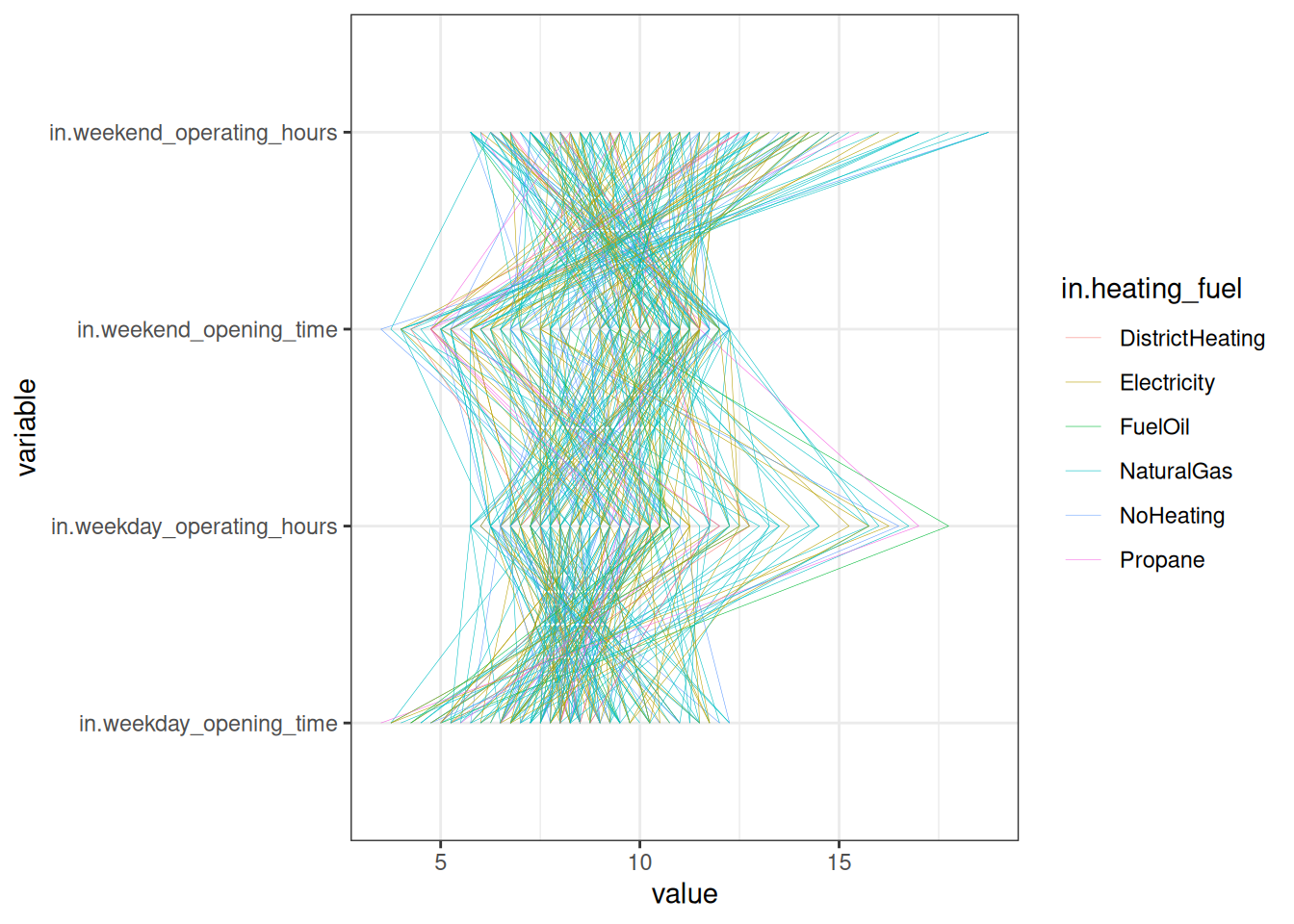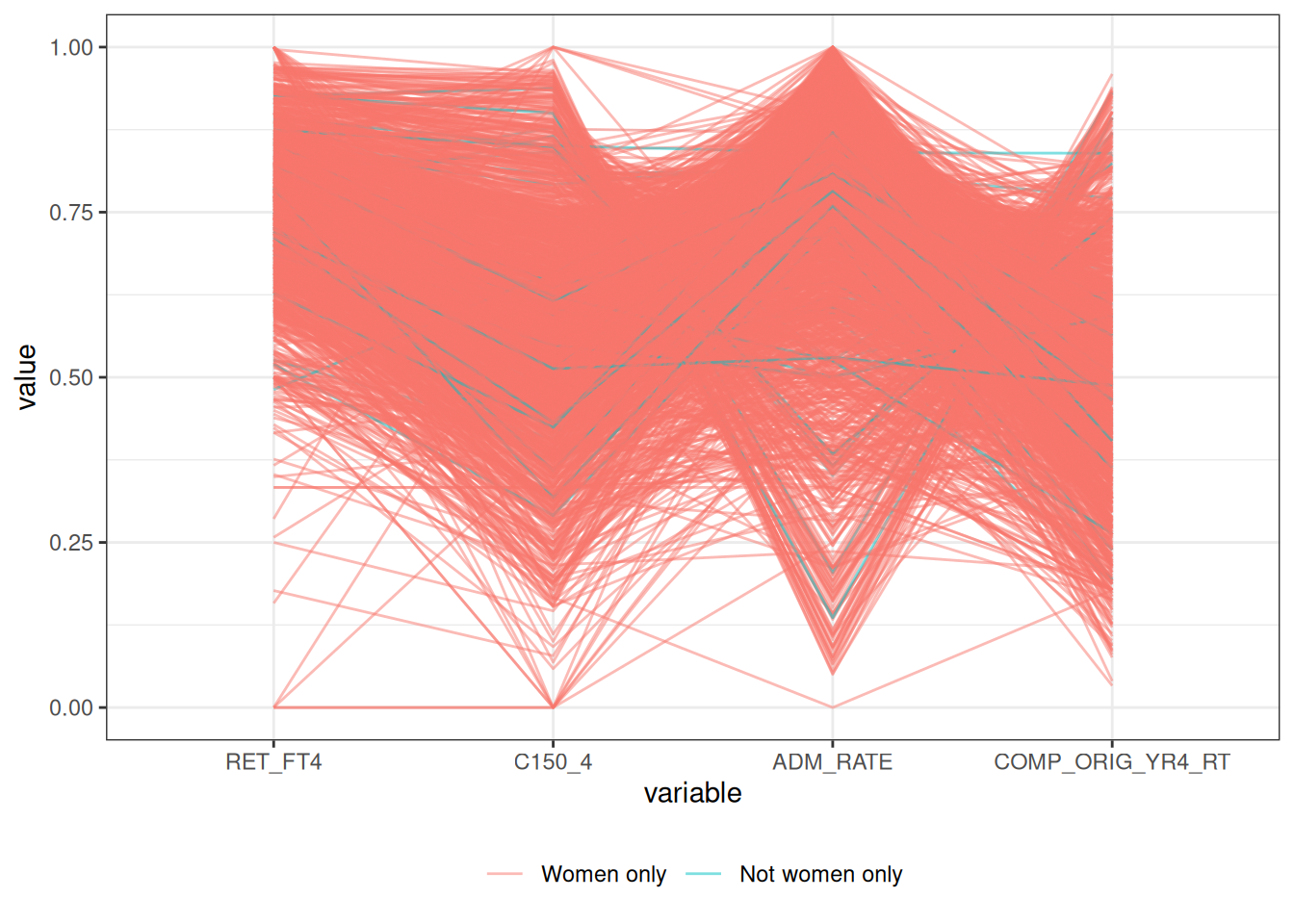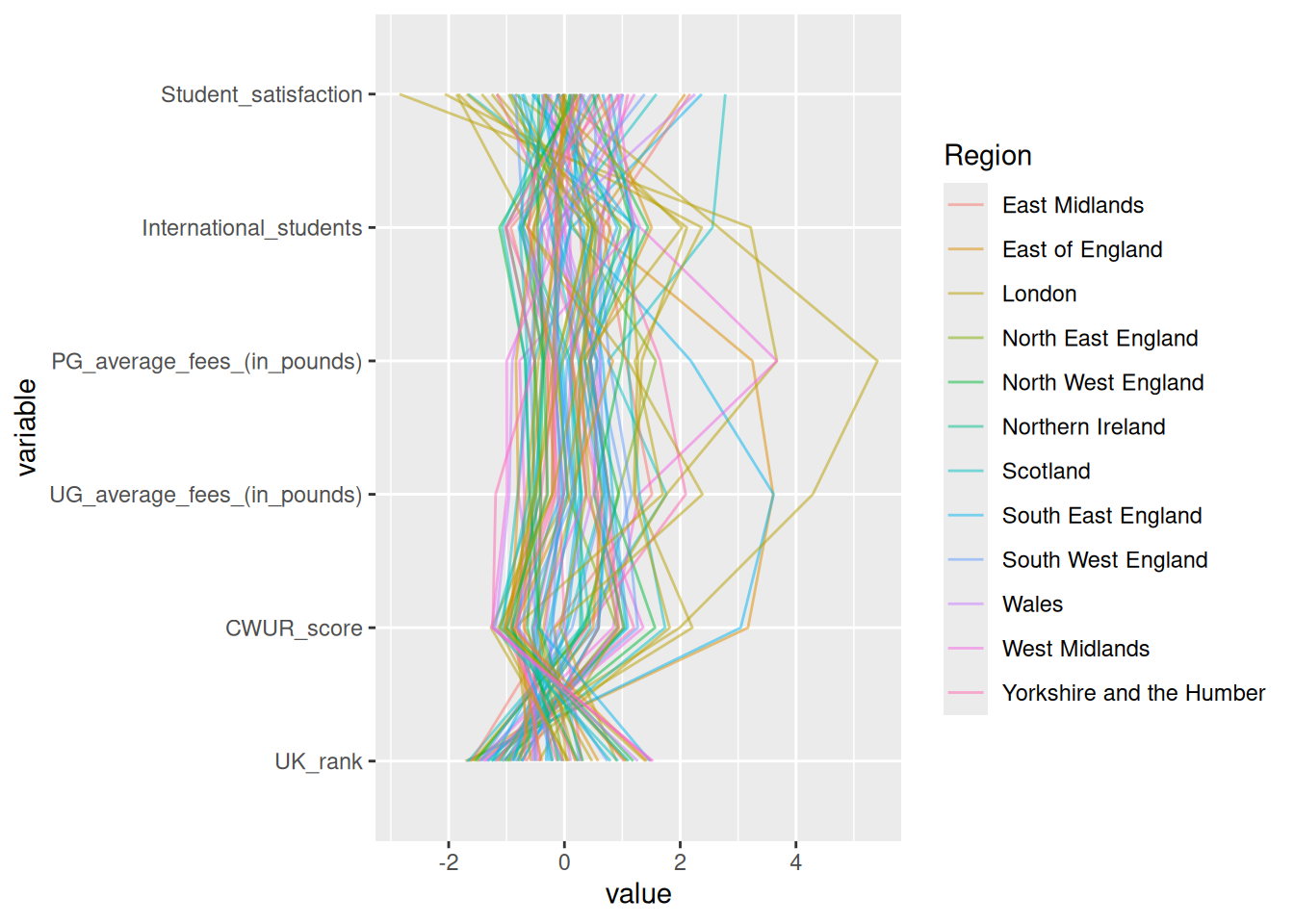# Chapter 18 Cutting room floor

It’s worth remembering that most graphs end up on the proverbial cutting room floor. Some graph types in particular are truly hit or miss: parallel coordinate plots are at the top of the list in this category. I’m including some of the “misses” here so you’ll realize you’re not alone if you create a graph that does not show anything worthwhile.

## 18.1 Parallel coordinate plots

``````library(dplyr)
library(forcats)
library(ggplot2)
library(stringr)
library(tibble)
library(tidyr)

oedi_building |>
filter(str_detect(in.building_type, "Office")) |>
GGally::ggparcoord(columns = 1:4, groupColumn = 5,
alphaLines = .5, splineFactor = 10)````````````oedi_building |>
select(c(starts_with("in.week")), in.heating_fuel) |>
rownames_to_column("ID") |>
pivot_longer(cols = starts_with("in.week"),
names_to = "variable", values_to = "value") |>
ggplot(aes(x = variable, y = value, group = ID, color = in.heating_fuel)) +
geom_line(lwd = .1) +
theme_bw() +
coord_flip()````````````# https://collegescorecard.ed.gov/data

df |>
na.omit() |>
mutate(COMP_ORIG_YR4_RT = as.numeric(COMP_ORIG_YR4_RT)) |>
mutate(WOMENONLY = fct_recode(factor(WOMENONLY), `Women only` = "0", `Not women only` = "1")) |>
GGally::ggparcoord(columns = 1:4, alphaLines = .5,
scale = "globalminmax", groupColumn = 5) +
theme_bw() +
theme(legend.position = "bottom", legend.title = element_blank())````````````uk <- read_csv("data/uk_universities.csv",
col_types = "ccdcddddddnncccccdddc")
uk |>
GGally::ggparcoord(columns = c(5, 7, 9:12),
alphaLines = .5, groupColumn = 2) +
coord_flip()````````````# https://www.strava.com/clubs/537620/leaderboard

run\$Longest <- parse_number(run\$Longest)
run\$Distance <- parse_number(run\$Distance)
run\$Pace <- parse_number(run\$`Avg. Pace`)
run\$Gain <- parse_number(run\$`Elev. Gain`)

run |>
filter(Distance < 1000, Rank <= 50) |>
select(Athlete, Distance, Runs, Longest, Pace, Gain) |>
parcoords::parcoords(rownames = F,
reorderable = TRUE,
brushMode = "1D-axes")``````
``````library(parcoords)
uk |>
filter(str_detect(Region, "England")) |>
select(University_name, UK_rank, UGfees = `UG_average_fees_(in_pounds)`,
PGfees = `PG_average_fees_(in_pounds)`,
International_students,
Student_satisfaction,
COL = `Estimated_cost_of_living_per_year_(in_pounds)`,
Campus_setting) |>
parcoords(rownames = FALSE,
reorderable = TRUE,
brushMode = "1D-axes",
color = list(colorBy = "Campus_setting",
colorScale = "scaleOrdinal",
colorScheme = "schemeCategory10"),
withD3 = TRUE,
width = 770,
height = 600)``````